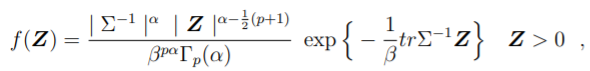# Multivariate Gamma Distributions

Share on

## What are Multivariate Gamma Distributions?

The Multivariate Gamma Distributions are generalizations of the univariate gamma distributions. Generally speaking, a random vector has a multivariate gamma distribution if it has gamma marginals .

There are dozens of different extensions of multivariate gamma distributions; some are defined by density functions, while others are defined by other formulas. However, the generalized multivariate gamma distribution probability density function can be defined as :Where Γpα is the multivariate gamma function.

## Types of Multivariate Gamma Distributions

Many different types are found in the literature, including:

• Dussauchoy and Berland’s  defined by a characteristic function,
• Gaver’s multivariate gamma distribution , which generates a multivariate distribution with a mixture of gamma variables with negative binomial weights.
• Krishnamoorthy and Parthasarathy’s  p-variate gamma distribution (also called the Wishart-chi-square distribution) exists for all positive integer degrees of freedom v and at least for all real values v > p-2, p ≥ 2.

## References

 Viraswami, K. (1991). On Multivariate Gamma Distributions. Retrieved January 1, 2022 from: https://escholarship.mcgill.ca/downloads/76537233r?locale=en
 Das, S. & Dey, D. On Bayesian Inference for Generalized Multivariate Gamma Distribution. Retrieved January 1, 2022 from: http://www2.stat.duke.edu/~sd83/Research/das-dey-mult-gamma.pdf
 Dussauchoy, A. & Berland, R. (1974). A multivariate gamma type distribution whose marginal laws are gamma, and which has a property similar to a characteristic property of the normal case. Statistical Distributions in Scientific Work, Vol. 1. Patil, G. et al (eds.). D. Reidel, Boston. 319-328.
 Gaver, D. P. (1970). Multivariate gamma distributions generated by mixture. Sankyhya Series A, 32, 123-126.
 Krishnamoorthy, A. S. and Parthasarathy, M., A multivariate gamma type distribution, Ann. Math. Stat. 22
(1951), 549-557.

CITE THIS AS:
Stephanie Glen. "Multivariate Gamma Distributions" From StatisticsHowTo.com: Elementary Statistics for the rest of us! https://www.statisticshowto.com/multivariate-gamma-distributions/
---------------------------------------------------------------------------Need help with a homework or test question? With Chegg Study, you can get step-by-step solutions to your questions from an expert in the field. Your first 30 minutes with a Chegg tutor is free!